# Maths - Category Theory - Yoneda

## Introduction

I tend to think of category theory as a way to define things from the outside (that is the fuctions/functors going in or out of it) in contrast set theory could be thought of a building up structures from inside. Yoneda gives us a way to link these two viewpoints.

So in category theory: we understand an object by its action on all other objects (or by the action of all other objects on it).

This leads us to the concept of 'representable functors' which gives us all the functors going into or out of an object.

## Yoneda Embedding

Yoneda allows us to relate categories to sets:

Category Theory Set Theory

Category theory models mathematics by arrows (morphisms/functors) between objects.

Higher order structures are given by arrows between arrows (natural transformations) and so on.Set theory models mathematics by the relationship between sets and their elements.

Higher order structures are given by sets within sets and so on.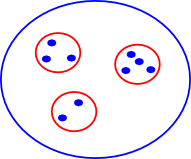The Yoneda embedding is an arrow between these.

### Generalising

We want to map morphisms to elements of a set. We can start with a mapping 'Q' which maps morphisms in a category 'C' to morphisms in Set.

We choose an object of C called 'A' and an element of Q(A) which we call 'q'.

So that, for all B:We can then use Q(b)(q) to link q to b.

 we have an isomorphism of sets: C(A,B) -> Q(B) C(A,-)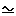Q which maps elements: b:A->B |-> Q(b)(q)

or equivalently an isomorphism of functors.

The functor Q:C -> Set is called representable.

## Covarient and Contravarient Functors

There are two ways that a given morphism can preserve structure:

 This is the covarient case where the start of the arrow in C maps to the start of the arrow in D and the end of the arrow in C maps to the end of the arrow in D.This is the contravarient case where the start of the arrow in C maps to the end of the arrow in D and the end of the arrow in C maps to the start of the arrow in D.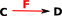Unless otherwise stated we usually assume that a morphism/functor is covarient so we can draw this as on the left.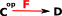For the contravarient case we could invent some sort of special symbol for a contravarient morphism/functor. However is usually easier to reverse the internal arrows in either C or D. On the left we have reversed C by making it Cop. So now we can use an ordinary arrow between C and D.

The way that covarient and contravarient functors arise, in this case, is as follows.

Let 'A', 'X' and 'Y' be elements of a class 'C'. let 'f' be a functor from 'X' to 'Y'. Assume that both 'A' and 'f' are fixed:

covarient contravarient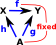• If 'f' and 'h' are known then we can derive 'g' (by composition)
• If 'f' and 'g' are known then we cannot derive 'h' (see concepts of section and retraction).

So given some fixed 'f' the 'g' depends on 'h' which I have drawn below as an arrow between arrows.

• If 'f' and 'g' are known then we can derive 'h' (by composition)
• If 'f' and 'h' are known then we cannot derive 'g' (see concepts of section and retraction).

So given some fixed 'f' the 'h' depends on 'g' which I have drawn below as an arrow between arrows.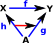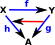This arrow goes in the same direction to f, hence it is covarient. This arrow goes in the opposite direction to f, hence it is contravarient.

We now need to combine these ideas with the concept of hom sets as follows.

## Hom Functors

For more information about homsets see the page here.

 Given a category with objects such as: a,b...x,y f:C(a,b) represents all the morphisms from a to b. g:C(x,y) represents all the morphisms from x to y. We can now take this up one level. A hom functor C(f,g) represents all the functors from f to g.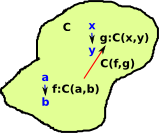We require this hom functor to interact nicely with the hom sets so the diagram on the left needs to commute.

This gives rise to a bi-functor to the category of sets:

Cop×CSet

A bifunctor is a functor in two arguments, it could be written H(Cop,C)Set . That is, it is contravarient in the first argument and covarient in the second).

## Representable Functors

(There are some good diagrams on ncatlab)

 Given a locally small category C, and an object C, the functor: HomC(C,−):C→Sets that sends objects to hom-sets and arrows f:A→B to functions: f*:HomC(C,A)→HomC(C,B) g |-> f•g is (or is isomorphic to) the Representable Functor.

In category theory we tend to work in terms of functors rather than objects, sometimes functors have more useful properties than objects. Representable Functors allow us to convert objects into functors. There are two ways to do this, either to or from a given object A.

covarient representable at A contravarient representable at ASo each element xX maps to a whole function f*:AX

where: X and A are objects in C, we think of A as being fixed.So each element xX maps to a whole function f*:XA. That is: 'f*' doesn't just map x to an element of A but it maps every element of X to A.

where: X and A are objects in C (or Cop so that we don't have to denote a covarient functor), we think of A as being fixed.

diagram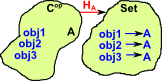We think of X as being variable, here shown as obj1, obj2, obj3...

f a is isomorphic to x -> a.

Example - An example of representable functors is powerset as described on this page.

## Covarient and Contravarient in Representable Functors

Here we combine the covarient and contravarient discussion above with representable functors to explain why representable functors come in covarient and contravarient types.

So far in representables we have mapped a element of an object to an element of a homset, but we want to know if this map preseves structure, so here we will see what happens when we map a whole function 'f':

covarient contravarient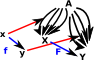From the triangle on the right of the diagram we have:

hom(A,Y) = f • hom(A,X)

that is post compose with f

So f maps to:

hom(A,X) -> hom(A,Y)

which is:

hom(A,X) -> f • hom(A,X)

From the triangle on the right of the diagram we have:

hom(A,X) = hom(A,Y) • f

that is pre compose with f

So f maps to:

hom(A,X) -> hom(A,Y)

which is:

hom(A,Y) • f -> hom(A,Y)

### Example 1 - Groups

 This is Cayleys theorom. A group is a simplified case because there is only one object. If we consider a group as a set with permutations (reversable functions) then there is only one object: G. So HomC(C,−) becomes G -> HomC(G,G). The Hom set is therefore the set of all the actions of the group which correspond to the elements of the group. So this is just the forgetful functor from the group to its underlying set.Here we want to relate permutations to other definitions of groups.

 So here we have a simple group, for example, in this case a cyclic group shown as a Cayley diagram:Here we are looking at functors between the structures which preserve structure.Here we apply one element of the group, in this case '2', as a representable. This must 'play well' with the internal structure of the group.If we repeat this for all permutations we get a set of permutations (homset).Cayley's theorem is more full dicussed on this page.

### Example 2 - Relating Homology and Cohomology

See the page here.

### Example 3 - Transforms in 3D Space using Quaternions

See the page here.

## Further Notation

Given any object AC there are two types of representable functors:

 HA = C(-,A) =Cop(A,-) is a contravarient representable at A HA =C(A,-) is a covarient representable at A

as follows:

contravarient representable at A covarient representable at A
type:

HA: Cop -> Set

this is a presheaf

HA: C -> Set
objects: X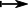C(X,A) XC(A,X)

where:

• C(,) is a hom-set of functors in C.

We are generating as set of functors to/from the object 'A'. The object 'A' is related to the concept of initial (covarient case) and terminal (contravarient case) objects.

A functor H: C -> Set is representable if:

• C and Set are small
• H preseves all small limits
• There exists a small set 'Set' of objects of C such that for any object cC and any element xH c there exists an sSet, an element yH s and an arrow f:s -> c with (H f)y = x.

So if objects in C map to functors in Set what happens to functors in C? We can represent them as natural transformations:

contravarient representable at A covarient representable at A
type: HA: Cop -> Set HA: C -> Set
objects:

XC(X,A)

HA = C(-,A) =Cop(A,-)

XC(A,X)

HA = C(A,-)

diagram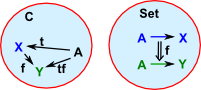Example in set.

So, for this example, we take C to be a set containing 3 elements: a, b and c.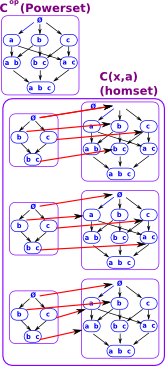Usually when working with natural transformations we can look at components in the codomain but, in the contravarient case, this is not very useful because everything maps to 'A'. So in this case C is like a component in the domain.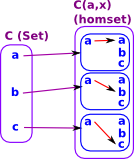Other examples:

• Group -> Cayley's theorem
• posets -> Upper sets

## Square Bracket Notation

[A,B] takes two categories A & B and produces a new category as follows: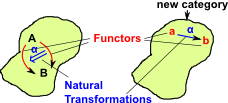The functors from A to B become the objects. The natural transformations become the arrows.

## Yoneda

So far given any AC we have:
AHA[Cop,Set]
this extends to a functor:
C[Cop,Set]

The arrows in the category are distinguished by their effect on generaised elements based at A. Such an object A is called a generator for C.

Looking at objects of C[Cop,Set] we have:

 AHA .| f \/ Hf natural transformation BHAHB

component of Hf at XC

(Hf)X: HAHB

 f _ C(X,A)C(X,B)

The Yoneda lemma, in any locally small category C then,

[Cop,Set](HA,X)X(A)

naturally in AC and X[Cop,Set]

Both the left and right sides are sets of natural transformations. [Cop,Set](HA,X) is the set of natural transformations in [Cop,Set] from HA to X.where: C is a category [Cop,Set] - Here we apply the above construction to Cop and Set. Cop is the dual of C, that is, all the arrows are reversed. For any object A of C there is a functor: HA: Cop -> Set (also written C(-,A)) this functor is defined on objects HA(B) = C(B,A) and on morphisms by HA(f) = f* (compose with f) X is a functor Cop -> Set For any category D and objects D,D' of D the set of morphisms from D to D' is written D(D,D')

## Example 1 - Groups

 When we represent a group as a permutation group each permutation (arrow) can be mapped onto a set element.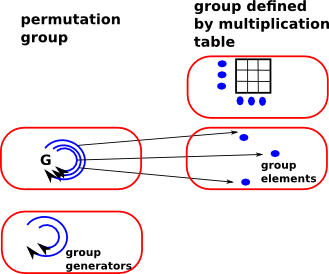The composition of the arrows with their associatively and identity then naturally map onto the group multiplication with its associatively and identity element.

## Example 2 - Sets

 We have two sets, 'A' and 'B', with an arbitrary mapping 'F' between them. We can define a subset of set 'B' by using the predicate 'P'. For every subset of 'B' defined by 'P' there exists a subset of 'A' defined by 'R'.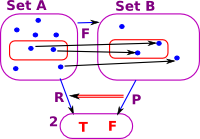However this does not necessarily work in the other direction. Here is an example of a subset of 'A' which does not map to a subset of 'B'.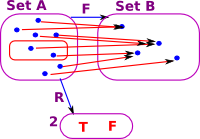So the map 'F' between objects induces a contravarient map between arrows P->R.

For more information about this relationship between sets and boolean logic see the page about topos theory.

## Program

Can we do somthing similar to the Yoneda embedding to a object oriented program? Here is a simple example written in 'Scala':

```class Complex(r: double, i: double) {
val real = r
val imag = i

def +(that: Complex) = new Complex(real + that.real, imag + that.imag)
def *(that: Complex) = new Complex(real*that.real - imag*that.imag,imag*that.real+real*that.imag)
}```

It has two values: real and imag (that I take to be like objects) and two operations: + and * (that I take to be like functors).

## Presheaf

A V-valued presheaf F on a category C is a functor F:Cop -> V

In the theory of topological space a sheaf is a tool for systematically tracking locally defined data attached to the open sets of a topological space.

More about presheaf in category theory on page here.

## Yoneda Proof

On site here is a proof written in Isabella.

metadata block
see also:

The following two videos discuss some of these concepts in a slightly topological way. For instance maps into sets related to fibres.

Some other background information.

Correspondence about this page

Book Shop - Further reading.

Where I can, I have put links to Amazon for books that are relevant to the subject, click on the appropriate country flag to get more details of the book or to buy it from them.The Princeton Companion to Mathematics - This is a big book that attempts to give a wide overview of the whole of mathematics, inevitably there are many things missing, but it gives a good insight into the history, concepts, branches, theorems and wider perspective of mathematics. It is well written and, if you are interested in maths, this is the type of book where you can open a page at random and find something interesting to read. To some extent it can be used as a reference book, although it doesn't have tables of formula for trig functions and so on, but where it is most useful is when you want to read about various topics to find out which topics are interesting and relevant to you.

Specific to this page here:

This site may have errors. Don't use for critical systems.

Copyright (c) 1998-2021 Martin John Baker - All rights reserved - privacy policy.